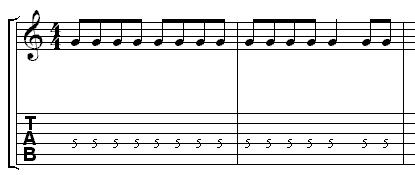# GuitarScholar.co.uk

## Time Signatures

The vertical lines on the staff are called bar lines. These separate the staff into different measures (also called bars). In figure 1 and figure 2 the time signatures are showing the amount and type of notes within each measure. Each measure in figure 1 contains four quarter notes. This is called 4/4 time. Each measure in figure 2 contains three quarter notes. This is called 3/4 time. Not all time signatures are in quarter notes.

Figure 1.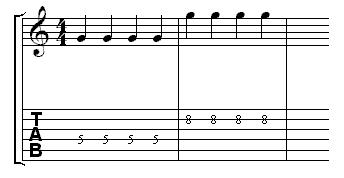Figure 2.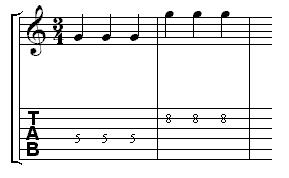Each measure in figure 3 contains twelve eighth notes. Each measure in figure 4 contains three half notes.

Figure 3.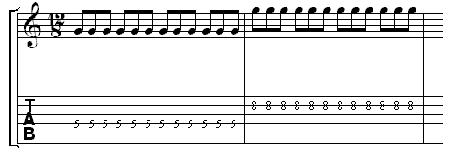Figure 4.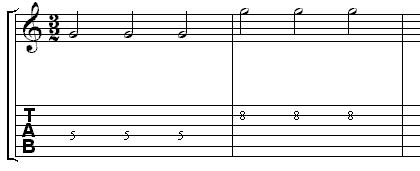A 4/4 time signature does not state that all of the notes have to be quarter notes. It just states that the total note duration in each bar has to add up to the equivalent of four quarter notes (see below).